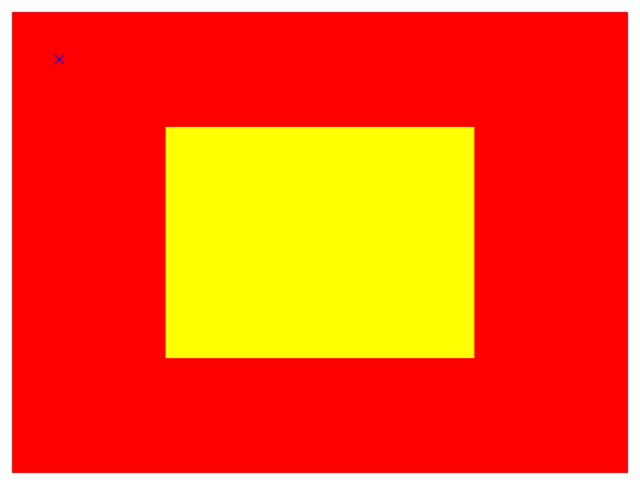Instance Method

# convert(_:to:)

Converts the point from the receiver’s coordinate system to the specified layer’s coordinate system.

## Parameters

`p`

A point specifying a location in the coordinate system of `l`.

`l`

The layer into whose coordinate system `p` is to be converted. The receiver and `l` must share a common parent layer. This parameter may be `nil`.

## Return Value

The point converted to the coordinate system of `layer`.

## Discussion

If you specify `nil` for the `l` parameter, this method returns the original point added to the layer's frame's origin.

Listing 1 shows code that creates two layers, `redLayer` and `yellowLayer`. `yellowLayer` is scaled so that it is half of its original size.

Figure 1 shows the two layers and an overlaid point (rendered as a blue cross) with a position of `(50.0, 50.0)` in the red layer's coordinate system.Figure 1 Layers with different coordinate systems

Listing 2 show how you can find the coordinates of that point in the yellow layer's coordinate system.

## See Also

### Mapping Between Coordinate and Time Spaces

`func convert(CGPoint, from: CALayer?) -> CGPoint`

Converts the point from the specified layer’s coordinate system to the receiver’s coordinate system.

`func convert(CGRect, from: CALayer?) -> CGRect`

Converts the rectangle from the specified layer’s coordinate system to the receiver’s coordinate system.

`func convert(CGRect, to: CALayer?) -> CGRect`

Converts the rectangle from the receiver’s coordinate system to the specified layer’s coordinate system.

`func convertTime(CFTimeInterval, from: CALayer?) -> CFTimeInterval`

Converts the time interval from the specified layer’s time space to the receiver’s time space.

`func convertTime(CFTimeInterval, to: CALayer?) -> CFTimeInterval`

Converts the time interval from the receiver’s time space to the specified layer’s time space